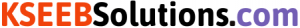# KSEEB Solutions for Class 6 Maths Chapter 8 Decimals Ex 8.3

Students can download Chapter 8 Decimals Ex 8.3 KSEEB Solutions for Class 6 Maths helps you to revise the complete syllabus.

## Karnataka State Syllabus Class 6 Maths Chapter 8 Decimals Ex 8.3

Question 1.
Which is greater?
a) 0.3 or 0.4
b) 0.07 or 0.02
c) 3 or 0.8
d) 0.5 or 0.05
e) 1.23 or 1.2
f) 0.099 or 0.19
g) 1.5 or 1.50
h) 1.431 or 1.490
i) 3.3 or 3.300
j) 5.64 or 5.603
Solution:
a) 0.3 or 0.4:-
The whole parts of these numbers are same. It can be seen that the tenth part of 0.4 is greater than that of 0.3 Hence, 0.4 > 0.3b) 0.07 or 0.02:-
Here, both numbers have same parts up to the tenth place However, the hundredth part of 0. 07 is greater than that of 0.02.

c) 3 or 0.8:-
It can be seen that the Whole part of 3 is greater than that of 0.8 Hence, 3 < 0.8

d) 0.5 or 0.05:- The Whole parts of these numbers are same. It can be seen that the tenth part of 0.5 is greater than that of 0.05, Hence 0.5 > 0.05.

e) 1.23 or 1.2:-
Here, both numbers have same parts up to the tenth place however, the hundredth part of 1.23 is greater than that of 1.20, Hence 1.23 > 1.20.

f) 0.099 or 0.19 :-
The whole parts of these numbers are same. It can be seen that the tenth parts of 0.19 is greater than that of 0.099 Hence, o.o99 < 0.19g) 1.5 or 1.50:-
Here, both numbers have the same parts up to the tenth place. Also, there is no digit at hundredth place of 1.5 . This implies that this digit will be 0, Which is same as the digit at the hundredth place of 1.50. Therefore, both these numbers are equal.

h) 1.431 or 1.490:- Here, both numbers have the same parts up to the tenth place, However, the hundredth part of 1.490 is greater than that of 1.431 Hence, 1.431 < 1.490

i) 3.3 or 3.300:- Here, both numbers have the same parts up to the tenth place. Also there is no digit at hundreth and thousandth place of 3.3 This implies that these digit are 0, Which are the same as the digit at the hundreth and thousandth place 3,300, Therefore both these number are equal Hence , 3.3 = 3.300 j) 5.64 or 5.603:- Here, both numbers have the same parts up to the tenth place, However the hundredth part of 5.64 is greater than that of 5.603
Hence, 5.640> 5603.

So, we see that if the digit at the thousandth place is the greatest, then the number formed is the greatest.